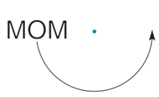Chapter 2.6, Problem 21E### Elementary Geometry for College St...

6th Edition
Daniel C. Alexander + 1 other
ISBN: 9781285195698

#### Solutions

Chapter
Section### Elementary Geometry for College St...

6th Edition
Daniel C. Alexander + 1 other
ISBN: 9781285195698
Textbook Problem
1 views

# What word is produced by 180 ° rotation about the point?To determine

To find:

The word produced by 180° rotation about the point.

Explanation

Given:

The given figure is,

Figure (1)

Definition Used:

Rotation about 360° means a full circle created, so rotation about 180° will create a semi- circle.

Approach:

Consider a reference point A on the word for rotation of word.

Rotate the word by some degrees at a time till semi-circle is created by rotation.

The rotation of figure is given below.

### Still sussing out bartleby?

Check out a sample textbook solution.

See a sample solution

#### The Solution to Your Study Problems

Bartleby provides explanations to thousands of textbook problems written by our experts, many with advanced degrees!

Get Started

#### Find more solutions based on key concepts

Elementary Technical Mathematics

#### (939592)1/2

Applied Calculus for the Managerial, Life, and Social Sciences: A Brief Approach

#### Write the sum in sigma notation. 12. 3+4+5+6+7

Single Variable Calculus: Early Transcendentals

#### Find for y defined implicity by .

Study Guide for Stewart's Multivariable Calculus, 8th